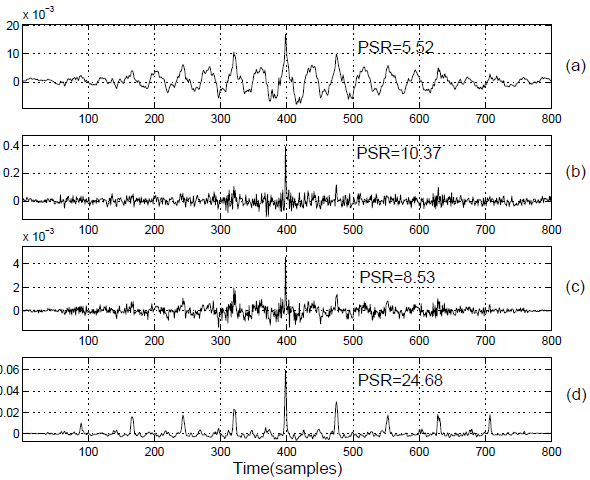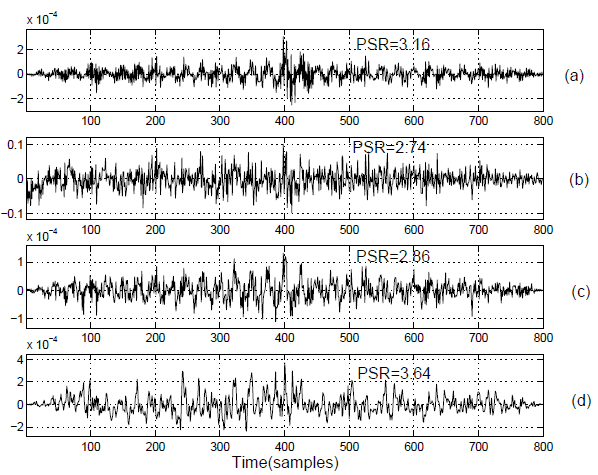2019-11-11  103 views 1

# 论文阅读整理（基于声源信息提取的说话人定位）

hat{s}(n)=-\sum\limits_{k=-1}^{p}{{{a}_{k}}s(n-k)}

e(n)=s(n)-\hat{s}(n)=s(n)+\sum\limits_{k=-1}^{p}{{{a}_{k}}s(n-k)}

A(z)=1+\sum\limits_{k=1}^{p}{{{a}_{k}}{{z}^{-k}}}

h(n)=\sqrt{{{e}^{2}}(n)+e_{h}^{2}(n)}

H(f)=-jsgn (f)## 来自外部的引用： 1 条

• 个人学习笔记整理 – 小奥の部落格